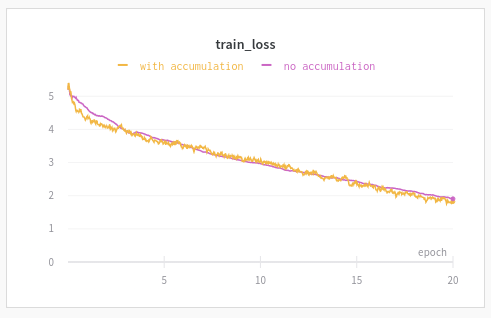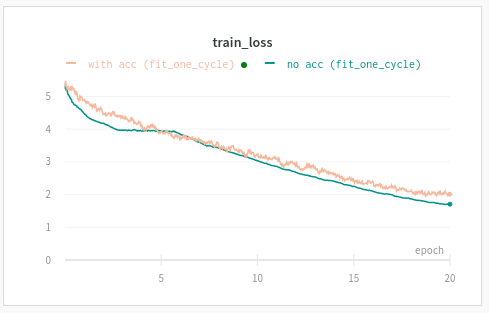# What is the v2 equivalent of "AccumulateScheduler"?

In v1, I subclassed `AccumulateScheduler` in order to implement gradient accumulation.

What would be the v2 approach to do the same?

Perhaps everything I need is available in the callbacks or gradient accumulation is already implemented somewhere in the framework??? Either way, definitely appreciate knowing which direction I should go in doing this with the v2 bits.

@wgpubs this was done in a very recent commit(like 12 hours ago).

3 Likes

Sweet! I knew it was wise to ask firstThanks!

UPDATE:

Wow … so much simpler in v2! Below, for posterity sake so folks know what this looked like in the old days, this is what I had to do:

``````class GradientAccumulation(AccumulateScheduler):
_order = -40 # needs to run before the recorder

def __init__(self, learn:Learner, n_step:int = 1, drop_last:bool = False):
super().__init__(learn, n_step=n_step, drop_last=drop_last)

self.acc_samples = 0
self.acc_batches = 0

def on_batch_begin(self, last_input, last_target, **kwargs):
"accumulate samples and batches"

self.acc_samples += last_input.shape
self.acc_batches += 1

def on_backward_end(self, **kwargs):
"accumulated step and reset samples, True will result in no stepping"
if (self.acc_batches % self.n_step) == 0:
for p in (self.learn.model.parameters()):
# wtg - not all params have a gradient here, so check for p.grad != None
self.acc_samples = 0
else:
return {'skip_step':True, 'skip_zero':True}

def on_epoch_end(self, **kwargs):
"step the rest of the accumulated grads if not perfectly divisible"
for p in (self.learn.model.parameters()):
# wtg - not all params have a gradient here, so check for p.grad != None

if not self.drop_last: self.learn.opt.step()
``````
3 Likes

Let me know how it works for you.
I just pushed it recently but I’m having a few issues on my project. There may be some incompatibility with fit_one_cycle and its scheduler but it could also be an issue with my own project.

Note:

• the callback in v2 is currently defined by number of samples needed (not number of batches)
• you will need to adjust your learning rate accordingly, ie if gradients are accumulated for 10 steps then you may want to divide your base learning rate (no accumulation) by 10

@sgugger I did the test you suggested previously.

• Gradient Accumulation: batch size divided by 10, update every 10 batches, learning rate divided by 10
• Training loop: regular `fit` (no `fit_one_cycle` for now to avoid potential issues with scheduler)
• 5 runs for baseline + 5 runs for gradient accumulation (we show mean and min/max for each group)

Results are consistently worse in the gradient accumulation variant (blue are the runs with and orange is the baseline).

As you can see, learning rate is fixed and has been divided by 10 while other optimizer parameters are the same.

When looking at gradients, they are about 10 times higher with `GradientAccumulation` (as expected) and weight parameters remain pretty much the same.

You can see the full results comparison here.

Do you have any idea of an other parameter I should adjust when doing gradient accumulation?
I was thinking it could be due to the weight decay but it is not called until all gradients have been accumulated…

Note: I’m going to propose a PR to `WandbCallback` which I modified so it now logs automatically some config parameters to help me make these graphs.

1 Like

Worked fine. Thanks much!

Just saw your post below … going to spend more time looking at your results. My use case was to fine tune a abstract summarization model where gradient accumulation was used by the paper’s authors (and not using one cycle). Will probably give the good ol’ fastai way a go to see how well it works itself.

1 Like

Good news, we can now perform tests and comparison easier through `WandbCallback` so I took advantage to revisit this topic.

Here is using the `fit` loop. The one with accumulation uses `bs=bs/10`, `GradientAccumulation(bs)`, `lr/10`Same thing with `fit_one_cycle`.I could have run the experiment a few more times and draw the mean & std of loss over experiment type but to me those results were already conclusive enough.

You can easily reproduce this experiment by running this notebook and looking at your W&B project page. Make sure to use “epochs” for your x-axis as there are more steps (batches) when you divide bs by 10.

4 Likes

Great job with the gradient accumulation, @boris

I don’t quite follow your posts though. Previously, you had plot that said gradient accumulation was not working as well as using a larger batch size, but your later post indicates it’s almost the same. What has changed? I see that the hyperparams for lr and bs are the same for both scenarios?

I also don’t follow the logic of why your bs in the Gradient Accumulation is smaller than the normal bs. You mentioned it counts samples, but not batch size, and I’m extremely confused by that statement (don’t understand what it means). If my batch size is 32 and the param I passed into Gradient Accumulation is 4, is the ‘effective batch size’ 32 x 4 = 128?

The only interpretation that makes sense with the examples provided is that batch size becomes ‘effective batch size’ when Gradient Accumulation is enabled? And the param passed into the callback is actually the actual batch size?

I had tested on a personal example and it was not yielding the results I expected initially but there could have been many reasons, probably though because of an issue in my own application as I was still discovering fastai2 which was evolving fast. The latest test shows it works as expected.

The way `GradientAccumulation` works is by accumulating the loss until a certain number of samples has been reached, then only perform back propagation.

If your batch size is 32 and the number of samples accumulated `n_acc` (parameter from `GradientAccumulation`) is 4, then it won’t perform anything special.
If you go the other way around with a batch size of 4 and `n_acc` of 32, then you will accumulate the loss for 32/4 = 8 batches prior to back propagation.

The only thing to be careful of is that when you do back propagation on one single batch, the loss of all the samples is averaged while `GradientAccumulation` will sum the losses from all your batches. It means that in the previous example, you could want to divide your learning rate by 8.
There could be other little impacts with Adam optimizers (and momentum) but I didn’t notice anything too sensitive there so it should be ok in most of cases.

2 Likes

Hello @boris,

I tried to use `GradientAccumulation` (with fastai v2) annouced by @muellerzr in a nlp learner (ie: learner with a language model as done in the notebook of @morgan that I fit with `fit_one-cycle()`). The loss is the normal cross-entropy loss for Language Model.

``````a = dsl.bs*k
``````

As you can see, my `n_acc` is a multiple (`k`) of the batch size (`bs`).

• When `k = 1` (no accumulating gradient), my learner works like it did not have the `cbs` with a training loss of about 2.
• When `k = 2` (accumulating gradient within 2 batches) or more, the training loss explodes (about 50 000) and decreases very slowly (ie, even after many weights updates of the model).

I saw that @wgpubs had this problem last year (but I guess, with fastai v1) but I don’t know if he resolved it.

Do you think that the class GradientAccumulation() does not work well with NLP models?
Thanks in advance to anyone with a suggestion.

I’m still with the same problem (training loss of 2 with bs = 32, and training loss of 50000 or more with GradientAccumulation of 64).

About the learning rate: my objective is to use LAMB optimizer with high LR and huge batch size (1000 or more). As I want to do that on just 1 GPU, I thought that the callback GradientAccumulation() was the solution.

My problem looks like a fastai v2 problem.

I just added the callback GradientAccumulation() in the notebook 10_nlp.ipynb: at the beginning of the epoch, the training loss is 18.978.176 instead of… 4 (bs = 128, n_acc = 256).

This is strange as it should be almost equivalent when you update the learning rate.

The main difference should be the effect of optimizers (momentum) or learning rate schedulers. Not sure if we could do something smart in the callback to account for it but it’s a bit tricky to think about what should be done here, even more when we can combine so many callbacks.

A few possible solutions:

• use SGD as an optimizer and `learn.fit` (instead of `fit_one_cycle`)
• maybe the issue is just at the start of training so you could train for an epoch (or a partial epoch) without gradient accumulation

Let me know how it goes. I’m curious if this can solve your problem!

Just do like me: in the notebook 10_nlp.ipynb of Jeremy, put `cbs=GradientAccumulation()` in `learn.fit_one_cycle()`. You should observe the huge running training loss (see my first screen shots)…

In fact, when you go until the end of the learner training (always the same example: the notebook 10_nlp.ipynb, see my second screen shot), you will observe that the valid loss and accuracy are right (compared to what Jeremy got) but the training loss is well high.

What I think:

• `GradientAccumulation()` works well.
• but… the running training loss up to the final one shows a true value but not the average one

How to correct the last point?

@pierreguillou There was a PR from @marii which may have fixed your issue: https://github.com/fastai/fastai/pull/3040

1 Like

@pierreguillou I notice you are using fp16 with gradient accumulation. I would expect this is your actual problem. I am moving on to look at getting native_to_fp16 working with gradient accumulation next. I will see if I can do a quick pass to check that to_fp16 is working with gradient accumulation.

fp16 has the concept of ‘loss_scaling’, which is artificially increasing the loss in order to avoid numbers too small for fp16 during backprop. I am guessing this loss scaled version is getting reported.

I expect your model is training correctly, because if the loss was actually that high in the beginning you would have no chance of getting a decent validation score.

If you would like I would appreciate it if you could create an issue on github with a link to this forum thread and a reproducible example(then @ me)

@pierreguillou You can follow this issue here: https://github.com/fastai/fastai/issues/3048

1 Like

It has already been merged, so feel free to try again